# What is Prime Factorization?

Did you know we have the power to change the way numbers are represented by using the prime factorization method?  Learn the prime factorization definition and how we use it in math.Author
Amber WatkinsPublished
November 7, 2023# What is Prime Factorization?

Did you know we have the power to change the way numbers are represented by using the prime factorization method?  Learn the prime factorization definition and how we use it in math.Author
Amber WatkinsPublished
Nov 7, 2023# What is Prime Factorization?

Did you know we have the power to change the way numbers are represented by using the prime factorization method?  Learn the prime factorization definition and how we use it in math.Author
Amber WatkinsPublished
Nov 7, 2023Key takeaways

• Prime factorization is one of the most fundamental ideas in math, and we use it often in our everyday lives without realizing it. It simply means breaking down a number using its prime factors.
• In fourth-grade math, we learn the foundational skills of factors and prime numbers, which help us to understand the fifth-grade math topic of prime factorization.
• Prime factorization also requires a good understanding of basic multiplication and division skills, which can be practiced on our math math app.

We all love chameleons. Why? Because they are able to change their appearance based on their environment. A chameleon can make itself blend in with a tree or leaves, and can even change into different colors.

Did you know we can do something similar with numbers? They can’t change into different colors or resemble a tree, but we can change the way they are represented in math depending on what we need them for.

One of the most common ways numbers can change the way they are represented is through prime factorization. What is prime factorization?

Prime factorization says that every number greater than 1 can be represented as the product of two or more prime numbers. That means numbers like 6, 15, all the way up to 100, and beyond can also be written as the product of prime numbers.

So how do we represent numbers using prime factorization, and is there only one way to do it? Let’s find out!

## Prime Factorization### Factors and Prime numbers

In order to understand Prime factorization, we need to understand two very important math terms: Prime numbers & Factors. Let’s review what those words mean together.

Factors are the numbers you multiply to get an answer.

For example, the factors of the number 5 are 1 and 5

Prime Numbers are any numbers that only have two factors. A good indicator that a number is prime, is if the only factors you can find are the number 1 and itself.

The first 10 prime numbers are 2, 3, 5, 7, 11, 13, 17, 19, and, 23.

We are going to keep these numbers handy to use later on in our practice of prime factorization.

Now let’s combine those two terms to understand the meaning of prime factorization. What is prime factorization?

### What is Prime Factorization?Prime factorization is a method used to break down a number using only prime numbers. When you use only prime numbers as factors they are called prime factors.

For example, the prime factors of 15 are 3 and 5, because they are both prime numbers.

Prime Factors of a Number

How to find the prime factors of a number? Use the list of prime numbers to make prime factorization easy for you!

Prime Number List:
2, 3, 5, 7, 11, 13, 17, 19, and, 23.

For example, what is the prime factorization of 12? If you look at the list of prime numbers, what combination of prime factors can you multiply to get 12?

____ x _____ x _____ = 12

Think to yourself: “3 x 2 equals 6. So now I’m halfway to 12. If I multiply it by another 2, I will end up with exactly 12.”

So, the prime factors of 12 are 2 x 2 x 3.

Rules when finding Prime Factorization
Remember, when finding the prime factorization of a number, it is okay to use the same factor more than once! Just make sure to put the numbers in order from least to greatest as your answer!

So when factoring the number 20 using prime factors, you may find 2 x 5 x 2. But when you put them in order from least to greatest you would write:

2 x 2 x 5 as the prime factorization of 20

### Prime Factorization MethodsThere are two main methods to find the prime factorization of a number. You can use the Factor Tree Method or the Division Method.

1. Factor Tree Method

The Factor Tree method of prime factorization starts with a large number and breaks it down until we reach the prime factors. Why is it called a Factor tree? Because all the numbers are connected with lines that look like the branches of a tree.

What is the prime factorization of 54?

First, find two factors that multiply to get 54.

9 x 5
Then, find two factors that multiply to get 9.
3 x 3
Next, find two factors that multiply to get 5.

1 x 5

Once all the factors are prime, you have found all the prime factors.

So, 3 x 3 x 1 x 5 = 54 is the prime factorization of 54.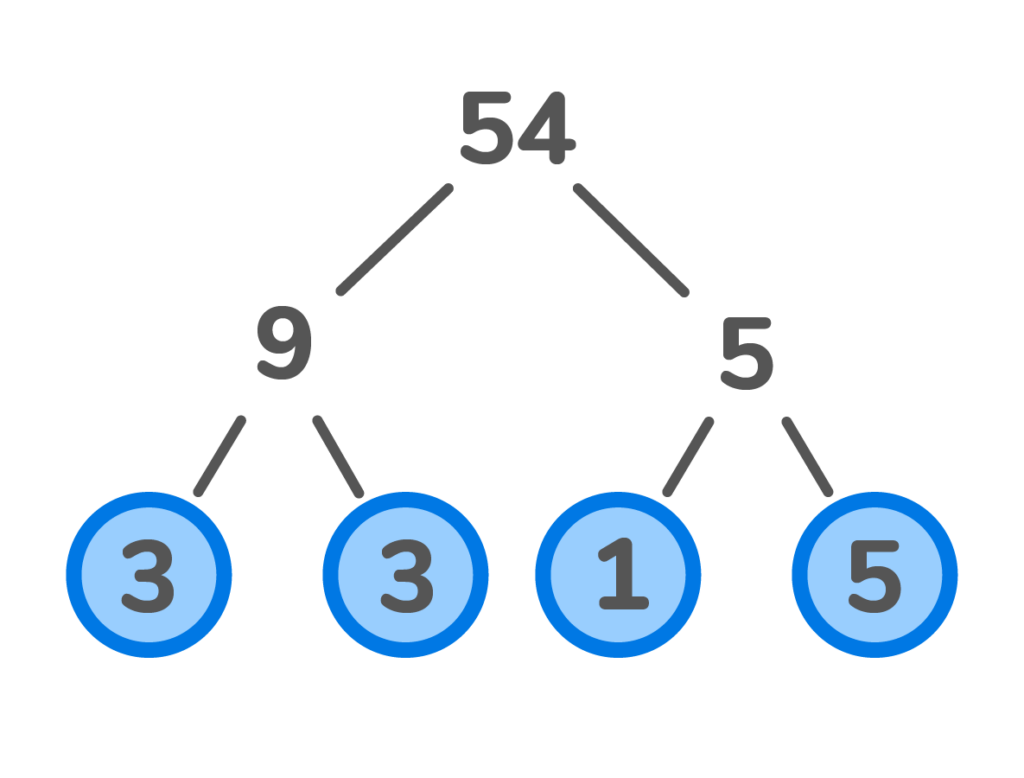2. Division Method

The Division Method uses the prime number list to guess and check which numbers can be divided into the number we are trying to factor. Let’s see how to use the Division Method of prime factorization.

What is the prime factorization of 49?

First, make a list of prime numbers.

Prime Number List:

2, 3, 5, 7, 11, 13, 17, 19, 23.

Next, start the process of guessing and checking to see which prime numbers can be divided into 49.

Can 49 be divided by the first prime number 2? No.
Can 49 be divided by the next prime number 3? No.
Can 49 be divided by the third prime number 5? No.
Can 49 be divided by the fourth prime number 7? Yes!

49 divided by 7 is 7. Because 7 and 7 are two prime factors, 7 x 7 is the prime factorization of 49.

### Prime Factorization in Real-Life ScenariosYou may be asking yourself, when do we use prime factorization in real life? Did you know there are many common examples where we use prime factorization, including when we are counting money in coins instead of dollars and also when we bake?

For example, if your cake recipe calls for 6 cups of flour. You may find it easier to count measuring 2 cups at a time. You would end up putting in 2 cups 3 different times.

2 x 3 is the prime factorization of 6

## Find Extra Practice that HelpsFinding the right math practice for your child can make a huge difference in the way they feel about math, especially when exploring more challenging topics such as prime factorization. Not only should you look for math practice that covers the topics your child will be exposed to in fourth and fifth grade, but it should also be created by professionals in Education.

Here at Doodle Learning, we have created the best math app for kids in grades k-5. The reason we can confidently say that is because our math app for kids covers the math standards for each grade and has been created by teaching professionals to make learning math fun and effective. While your child is solving the next few prime factorization problems, why not check out our app today?

## Prime Factorization Practice Problems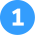What is the prime factorization of 36 using the factor tree method?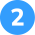What is the prime factorization of 24 using the factor tree method?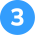What is the prime factorization of 48 using the division method?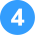What is the prime factorization of 22 using the division method?1. What is the prime factorization of 36 using the factor tree method?
1. Write the number 36 on top of the factor tree.
2. Underneath 36, find two numbers that multiply to equal 36, use 12 x 3. Circle the number 3 because it is a prime factor.
3. Since the number 12 is not a prime number, we find two numbers that multiply to equal 12, 4 x 3. Circle the number 3 because it is a prime factor.
4. Since the number 4 is not a prime number, we find two numbers that multiply to equal 4, 2 x 2. Circle both 2’s because they are prime factors.
5. Order the prime factors that were circled from least to greatest 2 x 2 x 3 x 3, which is the prime factorization of 36.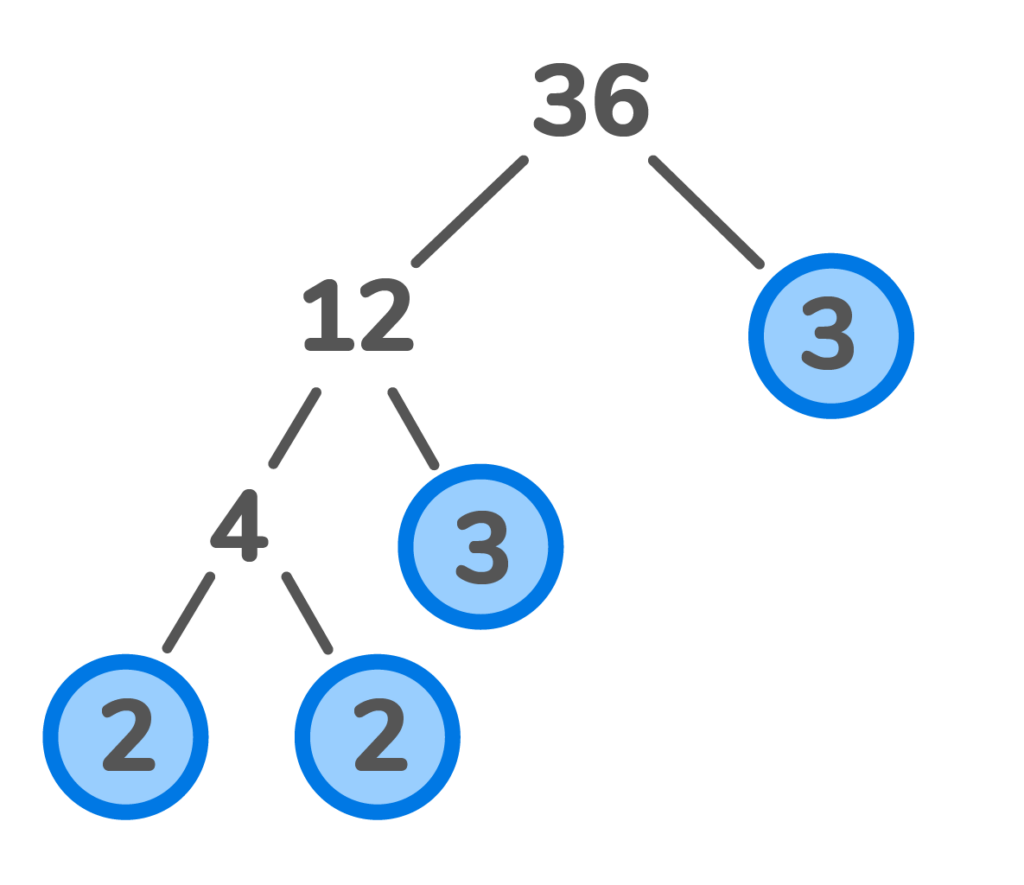2. What is the prime factorization of 24 using the factor tree method?

1. Write the number 25 on top of the factor tree.
2. Underneath the 24, find two numbers that multiply to equal 24, 12 x 2. Circle the 2 because it is a prime factor.
3. Since the number 12 is not a prime number, we find two numbers that multiply to equal 12, 6 x 2. Circle the number 2 because it is a prime factor.
4. Since the number 6 is not a prime number, we find two numbers to that multiply to equal 6, 3 x 2. Since they are both prime factors, we circle them both.
5. Order the prime factors that were circled from least to greatest 2 x 2 x 2 x 3, which is the prime factorization of 24.
1.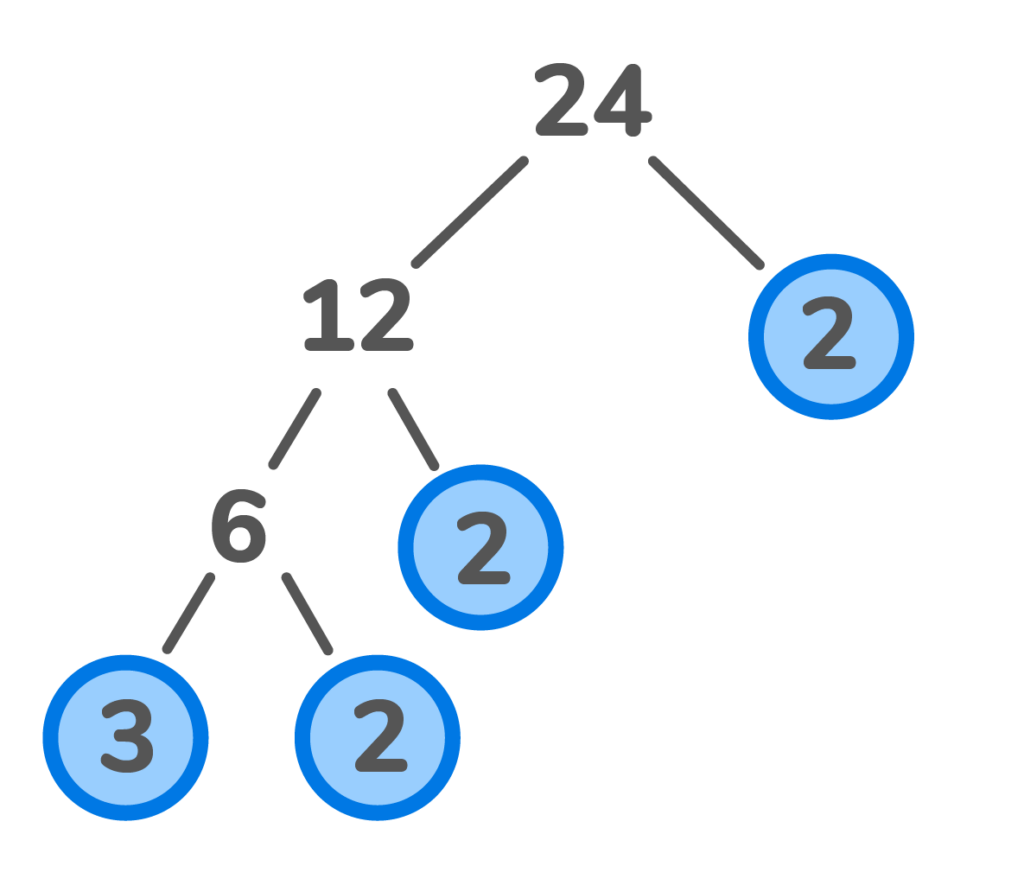3. What is the prime factorization of 48 using the division method?

1. Write a list of prime numbers  2, 3, 5, 7, 11, 13, 17, 19, 23.
2. Begin the process of Guess & Check:
1. Can 48 be divided by the first prime number 2? Yes. 48 divided by 2 is 24. Circle the number 2 because it is a prime factor.
2. Can the number 24 be divided by 2? Yes, 24 divided by 2 is 12. Circle the number 2 because it is a prime factor.
3. Can the number 12 be divided by 2? Yes, 12 divided by 2 is 6. Circle the number 2 because it is a prime factor.
4. Can the number 6 be divided by 2? Yes, 6 divided by 2 is 3. Circle the 2 and 3 because they are both prime factors.
5. Now order all the prime factors that were circled from least to greatest: 2 x 2 x 2 x 2 x 3 is the prime factorization of 48.

4. What is the prime factorization of 22 using the division method?

1. Write a list of prime numbers: 2, 3, 5, 7, 11, 13, 17, 19, 23.
2. Begin the process of Guess & Check:
1. Can 22 be divided by the first prime number 2? Yes, 22 divided by 2 is 11. Because 2 and 11 are prime factors, circle both of them.
2. Order the factors that were circled from least to greatest: 2 x 11 is the prime factorization of 22.Prime factorization in math is when you break down a composite number using only its prime factors.

The prime factors of a number are prime numbers you can multiply to get a number. Prime factors can only be prime numbers.

Prime factorization is important because one of the most important math theorems relies on it. This theorem states every number greater than 1 can be represented as the product of two or more prime numbers.

You use prime factorization in fourth and fifth grade, but also in everyday life. For example, you use prime factorization when counting money in coins instead of dollars. You also use prime factorization when baking with smaller measurements to add up to the total amount the recipe calls for.Lesson credits

Amber Watkins

Amber is an education specialist with a degree in Early Childhood Education. She has over 12 years of experience teaching and tutoring elementary through college level math. "Knowing that my work in math education makes such an impact leaves me with an indescribable feeling of pride and joy!"

Amber Watkins

Amber is an education specialist with a degree in Early Childhood Education. She has over 12 years of experience teaching and tutoring elementary through college level math. "Knowing that my work in math education makes such an impact leaves me with an indescribable feeling of pride and joy!"# Are you a parent, teacher or student?

Are you a parent or teacher?

## Hi there!

Book a chat with our team